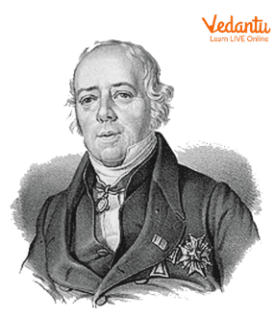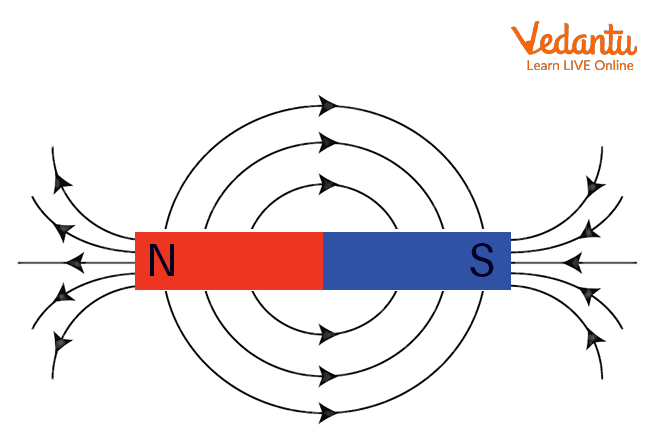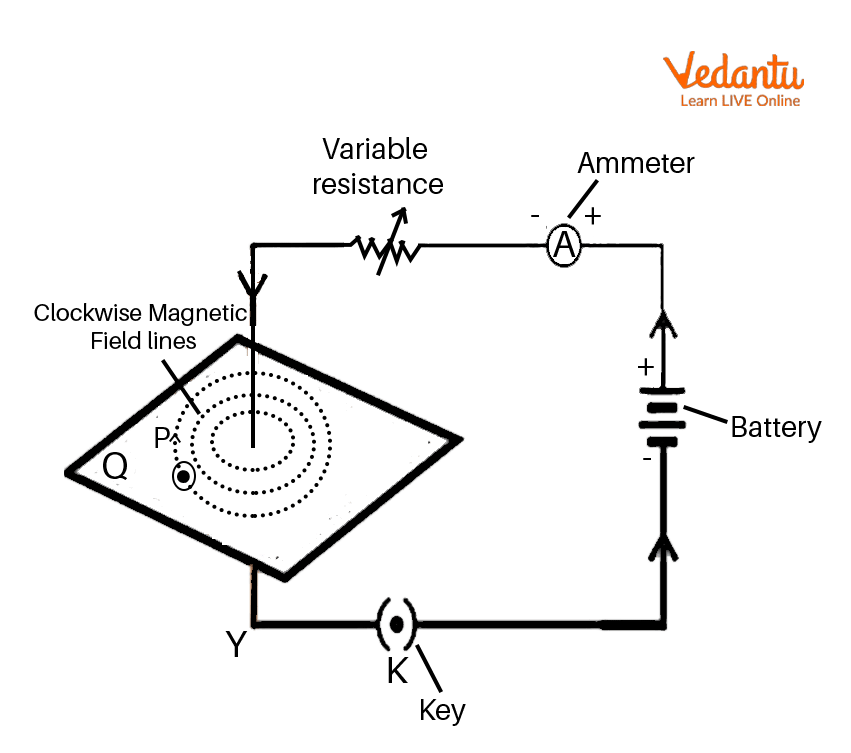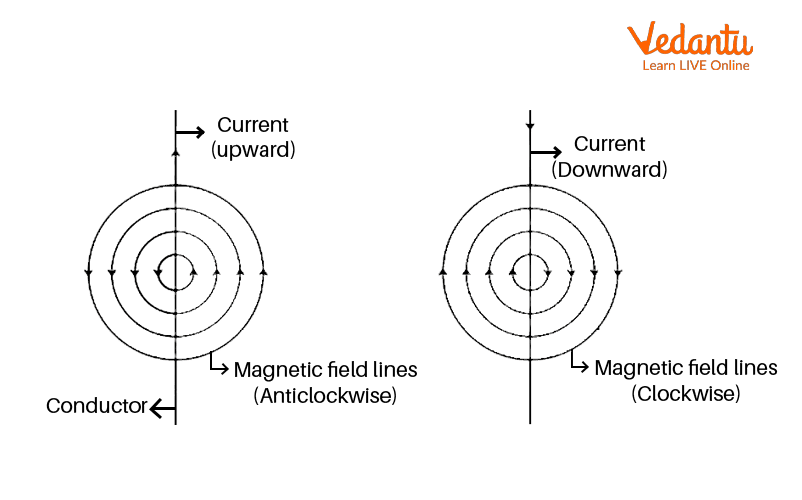Courses
Courses for Kids
Free study material
Free LIVE classes
More

# Magnetic Field Due to a Current Through a Straight ConductorLIVE
Join Vedantu’s FREE Mastercalss

## An Introduction to Magnetic Field and Current Through a Straight Conductor

With the help of magnetic field lines, we can visualise the magnetic field. Faraday introduced the concept of the magnetic field lines. The magnetic field lines give us the pattern of the magnetic field. Using iron filings or a magnetic compass, we can find the shape of the magnetic field.

In this article, we will have a deep insight into the magnetic field produced by a current carrying conductor and Maxwell’s right hand thumb rule to find the direction of the magnetic field.

## History of InventorHans Christian Oersted

Magnetism has been known since ancient times. In 1820, Hans Christian Oersted invented a very useful phenomenon. He was born in Rudkobing, Denmark. He observed that when the electric wire carries electric current, it behaves like a magnet. He established the relation between electricity and magnetism in the 19th Century.

## What is Magnetic Field?

The region surrounding the magnet in which the force of the magnet can be felt is called the extent of the magnetic field. Its SI unit is Tesla and it is named after the American Scientist Nikola Tesla. Gauss is the smaller unit of the magnetic field.

## Properties of Magnetic Field Lines

The list of properties of magnetic field lines of a bar magnet is as follows:Magnetic Field Lines

• Magnetic field lines generally originate from the North Pole of the magnet and end at the South Pole but inside the magnet, the magnetic field lines are directed from the South Pole to the North Pole. Thus, we can say that they are closed curves.

• These field lines can never intersect each other because at the point of intersection, we get two directions of magnetic field which is not possible.

• The magnetic field is strong where field lines are crowded and vice-versa.

## Magnetic Field Due to a Current in Straight Conductor

Consider the circuit shown here. Here, the conductor is connected to a simple circuit consisting of a variable resistance, an ammeter and a battery. The top end of the conductor is connected to the positive end of the battery. The other end of the conductor is connected to the negative side of the battery. The conductor is passed through a small sheet of cardboard and we have sprinkled some iron filings on the cardboard around the conductor.

As soon as we turn on the battery, the current starts flowing. As soon as the current starts flowing, we see that the iron filings which were randomly arranged around the conductor start arranging themselves in a specific pattern and the specific pattern is concentric circles which we have shown in the figure given below. All these concentric circles have just one centre which is nothing but the conductor itself and from the centre, the magnetic field originates in the form of concentric circles.Magnetic Field

We can find out the direction of the magnetic field with the help of Maxwell’s right hand thumb rule. This rule says that if you point the thumb in the direction of the current, then the direction in which your fingers curl the conductor will give you the direction of the magnetic field.

So, in order to apply the right hand thumb rule, hold a straight conductor in your right hand such that your thumb points the direction of current of this straight conductor, then the direction in which fingers are wrapped around this straight conductor is the direction of the magnetic field. This is shown in the below figure. This we can understand with the help of the figure given below.

If the conductor is carrying current in an upward direction, then the direction of the magnetic field will always be in an anticlockwise direction. But if the conductor is carrying current in a downward direction, then the direction of the magnetic field will be in a clockwise direction.Direction of Magnetic Field

If the direction of current is changed, the direction of magnetic field lines also changes which we can see in the above figure.

The magnitude of magnetic field produced by this straight current carrying conductor at a given point is

• Directly proportional to the current passing through this straight conductor. That means, $B$ is proportional to $I$

• Inversly proportional to its distance $r$ from this current carrying straight conductor. That means, $B$ is inversely proportional to $\dfrac{1}{r}$.

• So, magnetic field due to straight current carrying conductor (infinitely long) is given by

$B = \dfrac{{{\mu _0}I}}{{2\pi r}}$

Where, ${\mu _0} = 4\pi \times {10^{ - 7}}Tm{A^{ - 1}}$ and it is the permeability of free space, $I$ is the current flowing in the long straight conductor and $r$ is the distance of the magnetic field from that straight conductor.

### Interesting Facts

• Magnetite is the most magnetic natural metal on the Earth.

• The magnetic field of the Earth is 1000 times weaker than the bar magnet.

• We can not separate the North Pole and the South Pole of a magnet.

## Conclusion

From the above discussion, we can conclude that the magnetic field lines around a current carrying straight conductor are concentric circles whose centres lie on the conductor. Moreover, if the direction of the current in a straight conductor is known, then with the help of Maxwell's right hand thumb rule, we can find the direction of the magnetic field produced by it.

Last updated date: 24th Sep 2023
Total views: 94.8k
Views today: 2.94k

## FAQs on Magnetic Field Due to a Current Through a Straight Conductor

1. State the differences between a bar magnet and a solenoid.

Here are a few points of difference between a bar magnet and a solenoid:

• In a bar magnet, magnetic poles can not be changed. This means that polarity is fixed and can not be easily reversed. The magnetic poles in solenoid can be interchanged. In other words, we can say that polarity can be reversed by changing the direction of current in the case of the solenoid.

• The magnetism is permanent in the bar magnet. It is temporary in the solenoid.

• The strength of the magnetic field remains the same in the bar magnet. We can either increase or decrease the magnetic field strength in the solenoid.

2. State the differences between diamagnetic substances and paramagnetic substances.

Here are a few points through which we can show the difference between these two types of substances:

• Diamagnetic substances are feebly repelled by the magnet. Paramagnetic substances are feebly attracted by the magnet.

• When diamagnetic substances are placed in a non-uniform magnetic field, they have a tendency to move from the stronger to the weaker part of the magnetic field. When paramagnetic substances are placed in a non-uniform magnetic field, they tend to move from the weaker to the stronger part of the magnetic field slowly.

• There is no effect of temperature on diamagnetic substances. Paramagnetic substances depend on temperature.

3. Explain ferromagnetic substances and a few of their properties briefly.

Ferromagnetic substances are those substances which are strongly attracted by a magnet. Iron, nickel and cobalt are examples of ferromagnetic substances. Here are some properties of ferromagnetic substances.

• When ferromagnetic substances are placed in a non-uniform magnetic field, they tend to stick at the poles where the magnetic field is strongest.

• Ferromagnetic substances do not lose their magnetism on removal of external magnetic field. That means, they show permanent magnets.

• All ferromagnetic substances become paramagnetic above a temperature called Curie temperature.